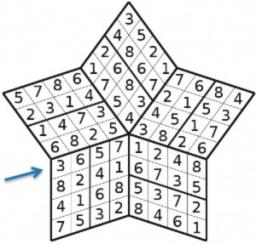# Probability 3065

Natural numbers 4,5,7,11,12 are given. Calculate the probability of the event that the sum of randomly selected three different numbers is less than 22.

p =  0.9

### Step-by-step explanation:Did you find an error or inaccuracy? Feel free to write us. Thank you!

Tips for related online calculators
Would you like to compute the count of combinations?